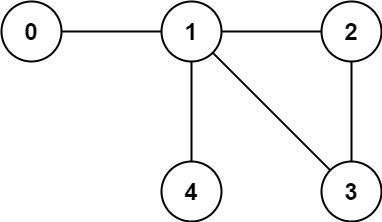# Graph Valid Tree LeetCode Solution

Difficulty Level Medium
TuSimpleViews 1889

## Problem Statement

Graph Valid Tree LeetCode Solution – Given the edges of a graph, check if the edges make up a valid tree. If yes, return true and false otherwise. The edges are given as a 2D array of size n*2

## Examples & Explanations

Example 1:```Input: n = 5, edges = [[0,1],[0,2],[0,3],[1,4]]
Output: true
Explanation: Since it does not conatain any cycle and all```

components are connected

`, it's a valid tree`

Example 2:```Input: n = 5, edges = [[0,1],[1,2],[2,3],[1,3],[1,4]]
Output: false
Explanation: False, because there exists a cycle```

## Approach

A graph is a valid tree iff

• does not consist of disconnected components
• does not have a cycle

### Algorithm

We will build the graph using a map and edges (2D array given as input). Check for any cycle present in the graph using DFS/BFS. We will mark the visited node as 1 and if we encounter the same node by traversing via its neighbors at some later point during processing, it means the graph contains a cycle.

Next, we will check if the graph contains any disconnected components. For this, we can check if any node remains unvisited. If there exists, it implies there are disconnected components.

## Code

### C++ Code for Graph Valid Tree

```class Solution {
bool isCyclic(map<int, vector<int>> &adj, vector<int>&vis, int v, int u) {
if(vis[v]) return 1;
vis[v]=1;
if(neighbor != u && isCyclic(adj, vis, neighbor, v))
return 1;
}
return 0;
}
public:
bool validTree(int n, vector<vector<int>>& edges) {
// contruct graph using map
for(auto &edge: edges) {
}

vector<int> vis(n,0);

return 0;

for(int i=0; i<n; i++) {
if(!vis[i])
return 0;
}

return 1;
}
};```

### Java Code for Graph Valid Tree

```class Solution {
private List<List<Integer>> adj = new ArrayList<>();
private Set<Integer> vis = new HashSet<>();

boolean dfs(int node, int parent) {
if (vis.contains(node)) return false;
for (int neighbour : adj.get(node)) {
if (parent != neighbour) {
boolean result = dfs(neighbour, node);
if (!result) return false;
}
}
return true;
}

public boolean validTree(int n, int[][] edges) {

if (edges.length != n - 1) return false;

for (int i = 0; i < n; i++)

for (int[] edge : edges) {
}

return dfs(0, -1) && vis.size() == n;
}

}```

## Complexity Analysis for Graph Valid Tree LeetCode Solution

Let E be the number of edges, and N be the number of nodes.

• Time Complexity: O(N + E)Creating the adjacency list of N nodes and E edges will take  O(E) + O(N) = O(N+E) time. As each node is added to the data structure only once, there will be N iterations and for each node, its adjacent edges will be traversed only once. We are ensuring this by keeping a visited array. Therefore, total E edges are iterated over once by the loop, and hence, the time complexity is O(N + E).
• Space Complexity: O(N + E)The adjacency list consists of N nodes with E edges, hence O(N + E) space. In the worst case, the stack/ queue will have all N nodes on it at the same time, resulting in a total of O(N) space. Hence the space complexity is O(E + N).
Translate »